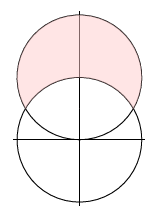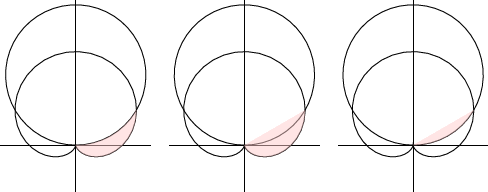$$\newcommand{\id}{\mathrm{id}}$$ $$\newcommand{\Span}{\mathrm{span}}$$ $$\newcommand{\kernel}{\mathrm{null}\,}$$ $$\newcommand{\range}{\mathrm{range}\,}$$ $$\newcommand{\RealPart}{\mathrm{Re}}$$ $$\newcommand{\ImaginaryPart}{\mathrm{Im}}$$ $$\newcommand{\Argument}{\mathrm{Arg}}$$ $$\newcommand{\norm}{\| #1 \|}$$ $$\newcommand{\inner}{\langle #1, #2 \rangle}$$ $$\newcommand{\Span}{\mathrm{span}}$$

# 10.3: Areas in Polar Coordinates

•• Contributed by David Guichard
• Professor (Mathematics) at Whitman College
$$\newcommand{\vecs}{\overset { \rightharpoonup} {\mathbf{#1}} }$$ $$\newcommand{\vecd}{\overset{-\!-\!\rightharpoonup}{\vphantom{a}\smash {#1}}}$$$$\newcommand{\id}{\mathrm{id}}$$ $$\newcommand{\Span}{\mathrm{span}}$$ $$\newcommand{\kernel}{\mathrm{null}\,}$$ $$\newcommand{\range}{\mathrm{range}\,}$$ $$\newcommand{\RealPart}{\mathrm{Re}}$$ $$\newcommand{\ImaginaryPart}{\mathrm{Im}}$$ $$\newcommand{\Argument}{\mathrm{Arg}}$$ $$\newcommand{\norm}{\| #1 \|}$$ $$\newcommand{\inner}{\langle #1, #2 \rangle}$$ $$\newcommand{\Span}{\mathrm{span}}$$ $$\newcommand{\id}{\mathrm{id}}$$ $$\newcommand{\Span}{\mathrm{span}}$$ $$\newcommand{\kernel}{\mathrm{null}\,}$$ $$\newcommand{\range}{\mathrm{range}\,}$$ $$\newcommand{\RealPart}{\mathrm{Re}}$$ $$\newcommand{\ImaginaryPart}{\mathrm{Im}}$$ $$\newcommand{\Argument}{\mathrm{Arg}}$$ $$\newcommand{\norm}{\| #1 \|}$$ $$\newcommand{\inner}{\langle #1, #2 \rangle}$$ $$\newcommand{\Span}{\mathrm{span}}$$

We can use the equation of a curve in polar coordinates to compute some areas bounded by such curves. The basic approach is the same as with any application of integration: find an approximation that approaches the true value. For areas in rectangular coordinates, we approximated the region using rectangles; in polar coordinates, we use sectors of circles, as depicted in $$\PageIndex{1}$$. Recall that the area of a sector of a circle is $$\alpha r^2/2$$, where $$\alpha$$ is the angle subtended by the sector. If the curve is given by $$r=f(\theta)$$, and the angle subtended by a small sector is $$\Delta\theta$$, the area is $$(\Delta\theta)(f(\theta))^2/2$$. Thus we approximate the total area as

$$\sum_{i=0}^{n-1} {1\over 2} f(\theta_i)^2\;\Delta\theta.$$

In the limit this becomes

$$\int_a^b {1\over 2} f(\theta)^2\;d\theta.$$

Example $$\PageIndex{1}$$: Area inside a cardiod

We find the area inside the cardioid $$r=1+\cos\theta$$.Figure $$\PageIndex{1}$$: Approximating area by sectors of circles.

Solution

$$\int_0^{2\pi}{1\over 2} (1+\cos\theta)^2\;d\theta= {1\over 2}\int_0^{2\pi} 1+2\cos\theta+\cos^2\theta\;d\theta= {1\over 2}\left.(\theta +2\sin\theta+ {\theta\over2}+{\sin2\theta\over4})\right|_0^{2\pi}={3\pi\over2}.$$

Example $$\PageIndex{2}$$: area between circles

We find the area between the circles $$r=2$$ and $$r=4\sin\theta$$, as shown in $$\PageIndex{2}$$.Figure $$\PageIndex{2}$$: An area between curves.

Solution

The two curves intersect where $$2=4\sin\theta$$, or $$\sin\theta=1/2$$, so $$\theta=\pi/6$$ or $$5\pi/6$$. The area we want is then $${1\over2}\int_{\pi/6}^{5\pi/6} 16\sin^2\theta-4\;d\theta={4\over3}\pi + 2\sqrt{3}.$$

This example makes the process appear more straightforward than it is. Because points have many different representations in polar coordinates, it is not always so easy to identify points of intersection.

Example $$\PageIndex{3}$$: area between curves

We find the shaded area in the first graph of $$\PageIndex{3}$$ as the difference of the other two shaded areas. The cardioid is $$r=1+\sin\theta$$ and the circle is $$r=3\sin\theta$$.Figure $$\PageIndex{3}$$: An area between curves.

Solution

We attempt to find points of intersection:

\eqalign{ 1+\sin\theta&=3\sin\theta\cr 1&=2\sin\theta\cr 1/2&=\sin\theta.\cr}

This has solutions $$\theta=\pi/6$$ and $$5\pi/6$$; $$\pi/6$$ corresponds to the intersection in the first quadrant that we need. Note that no solution of this equation corresponds to the intersection point at the origin, but fortunately that one is obvious. The cardioid goes through the origin when $$\theta=-\pi/2$$; the circle goes through the origin at multiples of $$\pi$$, starting with $$0$$.

Now the larger region has area

$${1\over2}\int_{-\pi/2}^{\pi/6} (1+\sin\theta)^2\;d\theta= {\pi\over2}-{9\over16}\sqrt{3}$$

and the smaller has area

$${1\over2}\int_{0}^{\pi/6} (3\sin\theta)^2\;d\theta= {3\pi\over8} - {9\over16}\sqrt{3}$$ so the area we seek is $$\pi/8$$.

## Contributors

• Integrated by Justin Marshall.